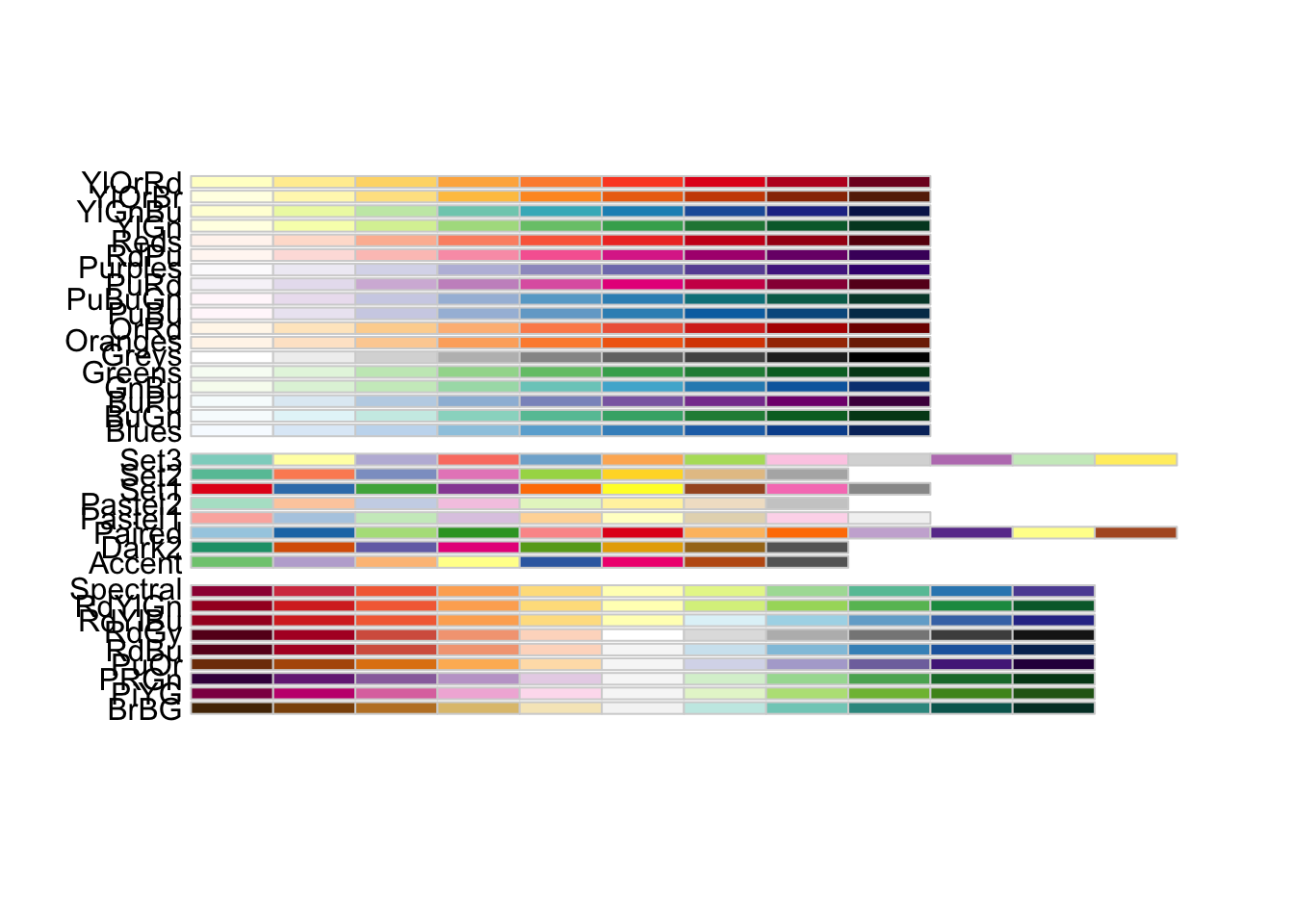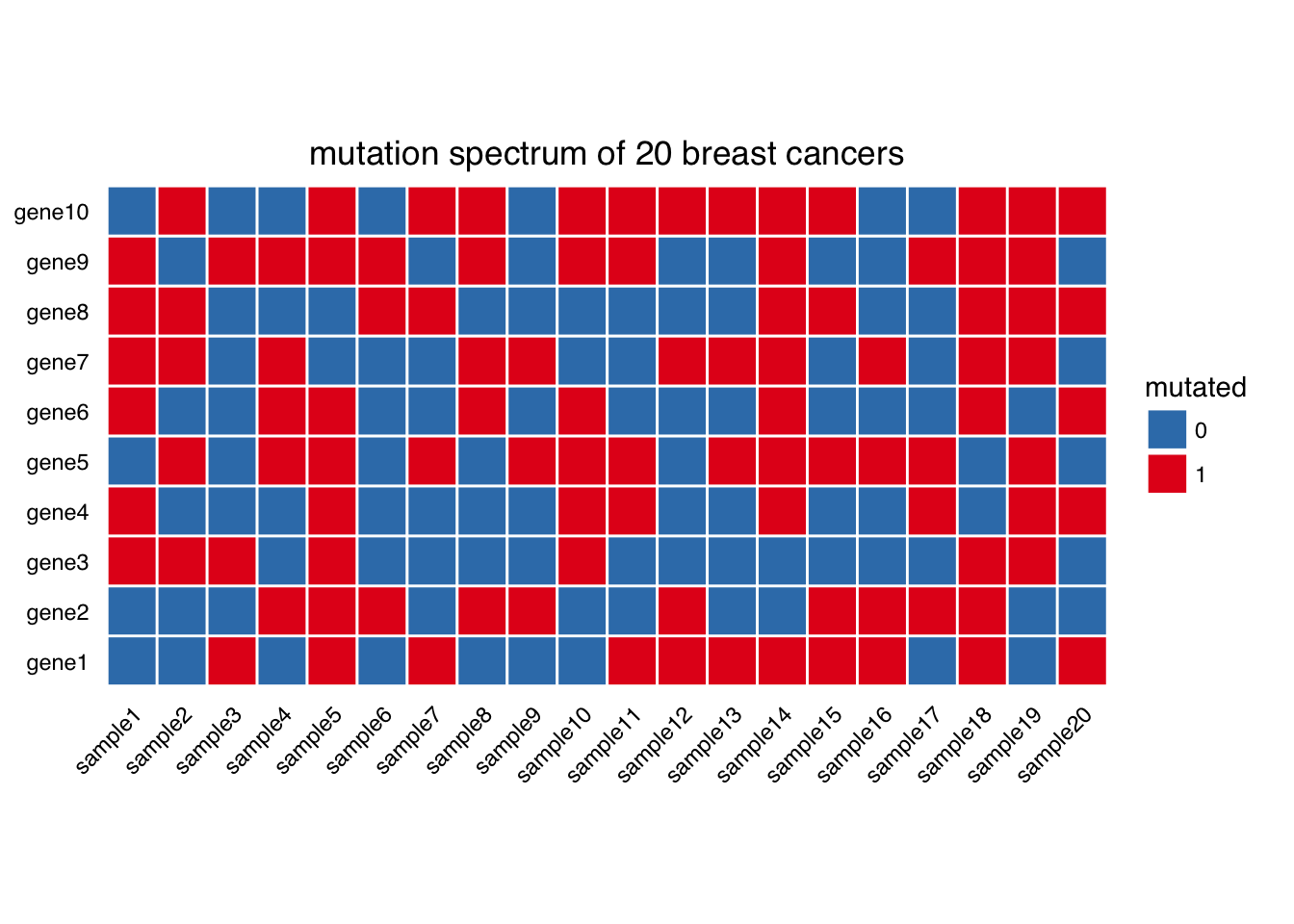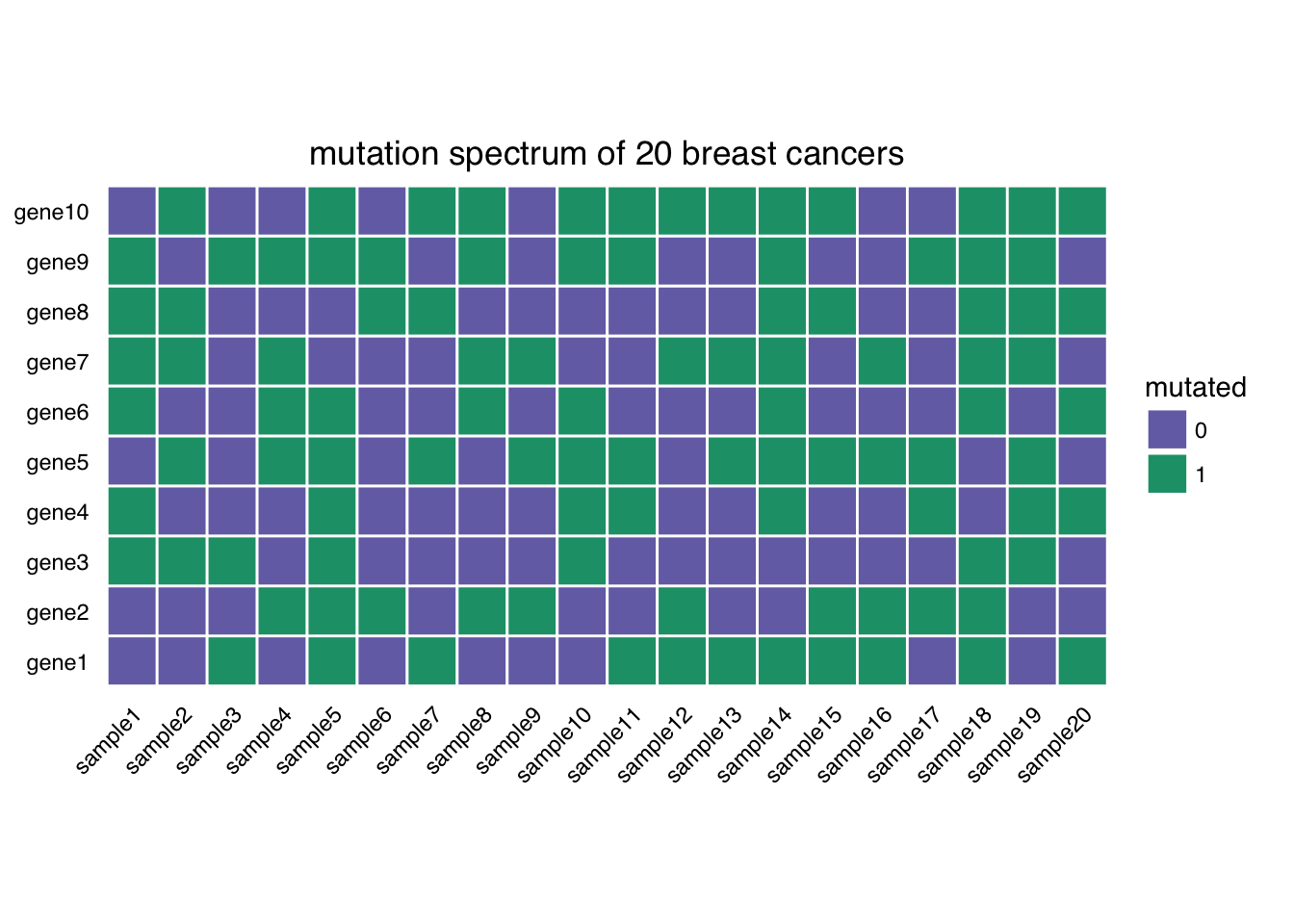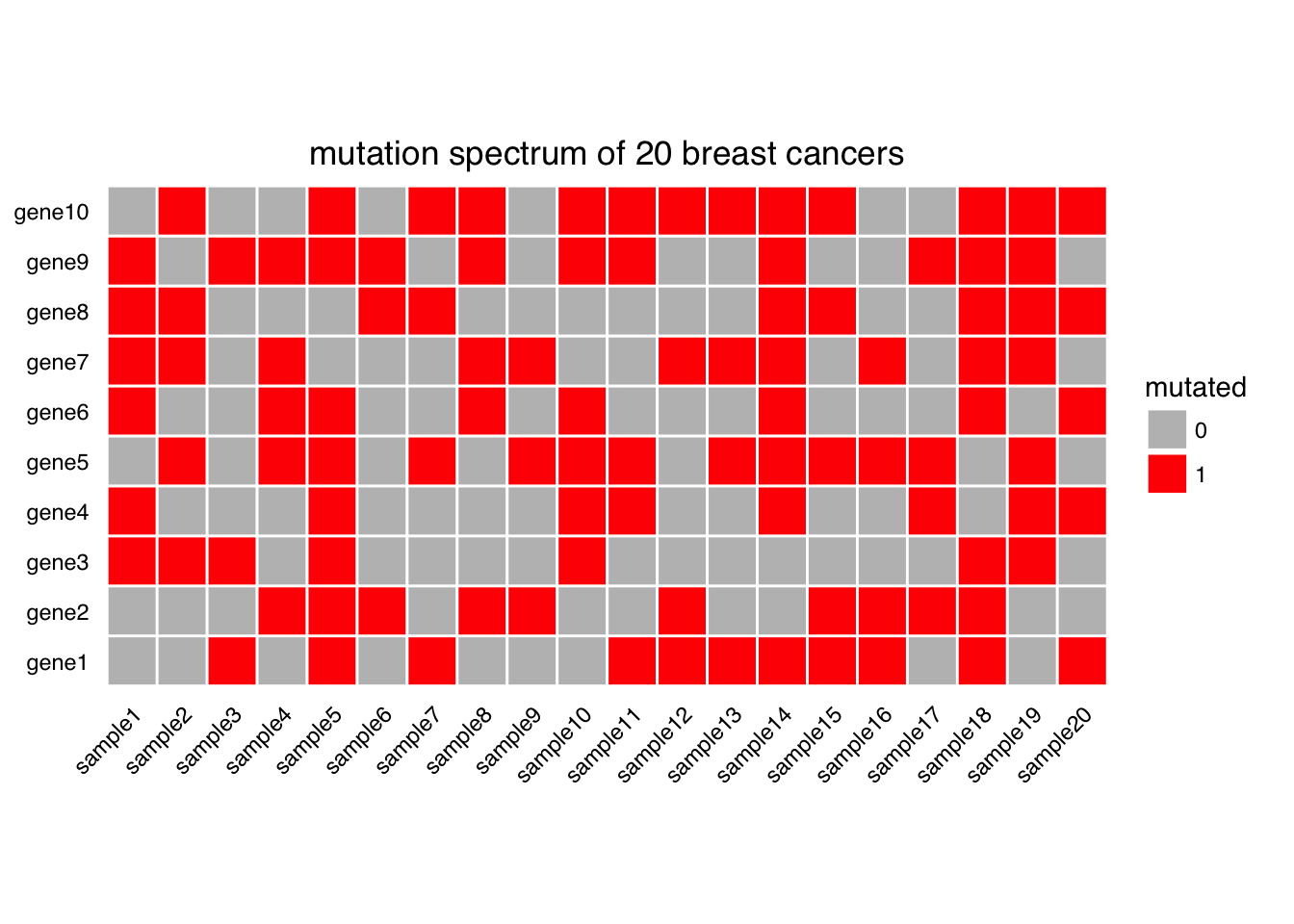## Wednesday, August 17, 2016

### Heatmap demystified part I: use heatmap to represent discrete values

In many Genomic papers, you will see heatmaps. Heatmaps are of no mystery. It is a way to visualize the data a.k.a. using colors to represent values. However, one really needs to understand the details of heatmaps. I recommend you to read Points of view: Mapping quantitative data to color and Points of view: Heat maps from a series of articles from Nature Methods.
Usually one has a matrix and then plot the matrix using functions such as `heatmap.2``pheatmap` or `Heatmap`.
I will start with a very simple using case for heatmap. We have sequenced 20 samples and identified mutations in 10 genes. some samples have the mutation in a certain gene, some samples do not have it. In this case, it will be a simple 0 (no mutation) or 1 (has mutation) to represent each data point. I am going to use`ggplot2` for this purpose, although the base R function `rect` can also draw rectangles.
Let’s simulate the data.
``````library(dplyr)
library(tidyr)
library(ggplot2)
set.seed(1)
# repeat the sampling
mut<- replicate(20, sample(c(0,1), 10, replace=TRUE))
mut<- as.data.frame(mut)
colnames(mut)<- paste0("sample", 1:20)
mut<- mut %>% mutate(gene=paste0("gene", 1:10))
``````##   sample1 sample2 sample3 sample4 sample5 sample6 sample7 sample8 sample9
## 1       0       0       1       0       1       0       1       0       0
## 2       0       0       0       1       1       1       0       1       1
## 3       1       1       1       0       1       0       0       0       0
## 4       1       0       0       0       1       0       0       0       0
## 5       0       1       0       1       1       0       1       0       1
## 6       1       0       0       1       1       0       0       1       0
##   sample10 sample11 sample12 sample13 sample14 sample15 sample16 sample17
## 1        0        1        1        1        1        1        1        0
## 2        0        0        1        0        0        1        1        1
## 3        1        0        0        0        0        0        0        0
## 4        1        1        0        0        1        0        0        1
## 5        1        1        0        1        1        1        1        1
## 6        1        0        0        0        1        0        0        0
##   sample18 sample19 sample20  gene
## 1        1        0        1 gene1
## 2        1        0        0 gene2
## 3        1        1        0 gene3
## 4        0        1        1 gene4
## 5        0        1        0 gene5
## 6        1        0        1 gene6``````
most of my codes follow a post Making Faceted Heatmaps with ggplot2
Tidy the data to the long format.
``````mut.tidy<- mut %>% tidyr::gather(sample, mutated, 1:20)

## change the levels for gene names and sample names so it goes 1,2,3,4... rather than 1, 10...
mut.tidy\$gene<- factor(mut.tidy\$gene, levels = paste0("gene", 1:10))
mut.tidy\$sample<- factor(mut.tidy\$sample, levels = paste0("sample", 1:20))``````
when fill the tiles with color, in this case, it is 0 or 1 discrete value. R thinks `mutated` is a numeric continuous value, change it to factor.
``````mut.tidy\$mutated<- factor(mut.tidy\$mutated)

## use a white border of size 0.5 unit to separate the tiles
gg<- ggplot(mut.tidy, aes(x=sample, y=gene, fill=mutated)) + geom_tile(color="white", size=0.5)``````
``````library(RColorBrewer) ## better color schema

## check all the color pallete and choose one
display.brewer.all()``````mutated will have color red, unmutated have color blue.
``gg<- gg + scale_fill_brewer(palette = "Set1", direction = -1)``
`geom_tile()` draws rectangles, add `coord_equal` to draw squres.
``````gg<- gg + coord_equal()

gg<- gg + labs(x=NULL, y=NULL, title="mutation spectrum of 20 breast cancers")

library(ggthemes)
##starting with a base theme of theme_tufte() from the ggthemes package. It removes alot of chart junk without having to do it manually.
gg <- gg + theme_tufte(base_family="Helvetica")

#We don’t want any tick marks on the axes

gg <- gg + theme(axis.ticks=element_blank())
gg <- gg + theme(axis.text.x=element_text(angle = 45, hjust = 1))
gg``````If you want to mannually fill the color, you can use `scale_fill_manual`, and check http://colorbrewer2.org/ to get the HEX representation of the color.
``````ggplot(mut.tidy, aes(x=sample, y=gene, fill=mutated)) + geom_tile(color="white", size=0.5) +
coord_equal() +
labs(x=NULL, y=NULL, title="mutation spectrum of 20 breast cancers") +
theme_tufte(base_family="Helvetica") +
scale_fill_manual(values = c("#7570b3", "#1b9e77")) +
theme(axis.ticks=element_blank()) +
theme(axis.text.x=element_text(angle = 45, hjust = 1))````````````ggplot(mut.tidy, aes(x=sample, y=gene, fill=mutated)) + geom_tile(color="white", size=0.5) +
coord_equal() +
labs(x=NULL, y=NULL, title="mutation spectrum of 20 breast cancers") +
theme_tufte(base_family="Helvetica") +
scale_fill_manual(values = c("gray", "red")) +
theme(axis.ticks=element_blank()) +
theme(axis.text.x=element_text(angle = 45, hjust = 1))      ``````Note that in a real genomic experiment, tens of thousands of genes will be assayed, and one can use tools such as `CoMET` to find the mutual exclusive mutations and plot as I just did. There is a so called oncoprint in many papers and essentially they are doing the same thing as I did here, but adding many details. see one example from `ComplexHeatmap`:
In this example, I showed an example of using heatmap to represent discrete values (yes or no mutation), in my following post, I will post how to use heatmap to represent continuous values and do clustering on rows and columns to find patterns (unsupervised clustering). `ggplot2` it self does not have clustering built-in. We will have to use the functions I mentioned in the begining of this blog. There are three main points I will stress on plotting a bi-clustered heatmap: